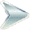We Can Figure This Out.org Virtual Lab: Scanning Electron Microscope (SEM)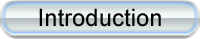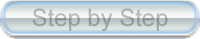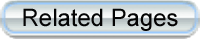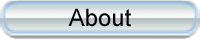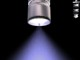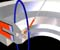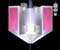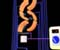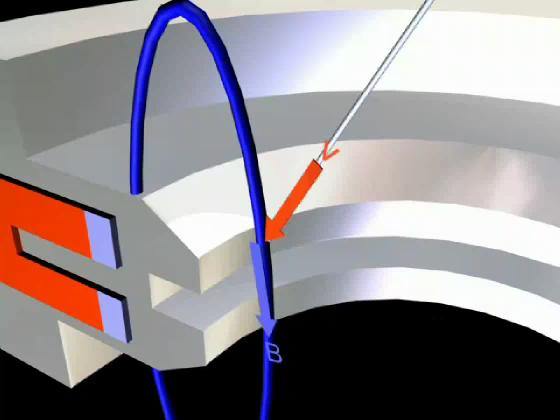© 2003-Present, John C. Bean

 In the force law, the "x" denotes a vector "cross-product." This means that we multiply the magnitude of v only by the component of B perpendicular to it. So here we divide the total magnetic field into components parallel and perpendicular to the electron's velocity. We then discard the parallel component because this creates no force on this electron.Last Scene Next Scene